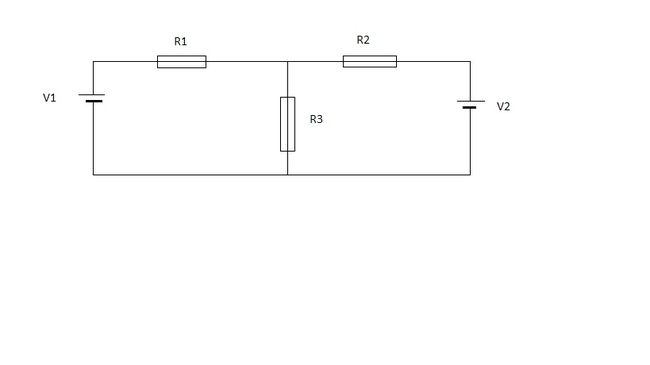# A Kirchhoff Voltage and Current Law problem.Find the voltage across the 40 ohm resistor.

Following values are to be used: $R_1=10 \Omega, R_2=20 \Omega, R_3 = 40 \Omega, V_1 = 10 V,V_2 = 20V$.

Answer is to be given in volts.

×

Problem Loading...

Note Loading...

Set Loading...